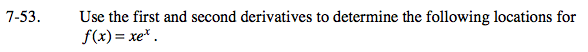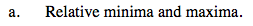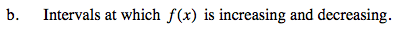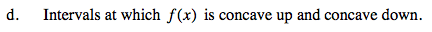### Home > CALC > Chapter 7 > Lesson 7.1.5 > Problem7-53

7-53.Remember: Finding where f '(x) = 0 or f '(x) = DNE will identify CANDIDATES for minima and maxima.
You need to complete the 1st or 2nd Derivative Test to confirm which is which.When f '(x) is positive, then f(x) has positive slopes, which means f(x) is increasing.Remember: Finding where f ''(x) = 0 or f ''(x) = DNE will identify CANDIDATES for inflection points.
You need to do further investigation to determine if it is (or is not) an inflection point.

Either test for a sign change of f ''(x) before and after the candidate point. Or evaluate f '''(x) at the candidate.
If f '''(candidate) ≠ 0, then it is a point of inflection.When f ''(x) is positive, then f '(x) has positive slopes, which means f(x) is concave up.